# Physical Chemistry : Other Atomic Concepts

## Example Questions

### Example Question #1 : Other Atomic Concepts

Which of the following represents the alkaline earth metal with the greatest atomic number?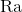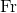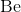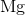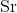Explanation:

Alkaline earth metals are group II elements; among them, radium has the largest atomic number.

### Example Question #1 : Other Atomic Concepts

Which of the following isotopes has the most neutrons?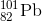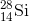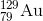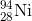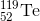Explanation: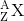this notation is commononly used in chemistry where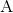represents the mass number, and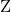represents the atomic number of element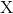.

To find the number of neutrons in each isotope, subtract the atomic number from the mass number.

Thus for, the number of neutrons is 67. This isotope has the most neutrons among the answer choices.

### Example Question #1 : Other Atomic Concepts

How many protons, neutrons and electrons are there in a neutral atom of the copper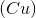isotope represented by a mass number of 65 and an atomic number of 29?

Protons: 29

Neutrons: 29

Electrons: 29

Protons: 36

Neutrons: 29

Electrons: 29

Protons: 29

Neutrons: 36

Electrons: 29

Protons: 29

Neutrons: 29

Electrons: 36

Protons: 36

Neutrons: 36

Electrons: 36

Protons: 29

Neutrons: 36

Electrons: 29

Explanation:

The number of protons for any atom is the same as the atomic number on the periodic table. Therefore, any isotope of copper will have 29 protons. Since the atom is neutral, the number of protons must be equal to the number of electrons. To find the number of neutrons, subtract the number of protons from the mass number to get 36 neutrons.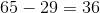### Example Question #62 : Nuclear, Quantum, And Molecular Chemistry

An ion from a given element has 13 protons and 10 electrons. What is the symbol of the ion?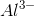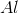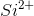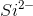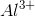Explanation:

The charge on the ion is equal to the difference between the number of protons and electrons: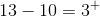The element has 13 protons, which means its atomic number is also 13. Thus this element is aluminum.

### Example Question #1 : Other Atomic Concepts

How many protons, neutrons and electrons are there in a neutral atom of the isotope of lithium-6?

Protons: 6

Neutrons: 6

Electrons: 3

Protons: 3

Neutrons: 3

Electrons: 3

Protons: 6

Neutrons: 3

Electrons: 6

Protons: 6

Neutrons: 3

Electrons: 3

Protons: 3

Neutrons: 6

Electrons: 3

Protons: 3

Neutrons: 3

Electrons: 3

Explanation:

The number of protons is equal to the atomic number on the periodic table. For lithium, this number is 3. Since this isotope is neutral, the number of electrons must be equal to the number of protons. To find the number of neutrons, subtract the number of protons from the mass number to obtain 3. The notation "lithium-6" indicates that the mass number of this isotope is 6.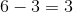### Example Question #1 : Other Atomic Concepts

An ion of a given element has 35 protons and 36 electrons. What is the symbol of the ion?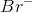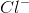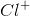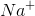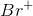Explanation:

The charge on the ion is equal to the difference between the number of protons and electrons.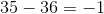The element has 35 protons, thus its atomic number is 35, which corresponds to bromine.

### Example Question #1 : Other Atomic Concepts

The __________ Principle states that electrons fill lower energy levels before filling higher energy levels and __________ Rule says that electrons fill electron orbitals within a subshell singly if possible before pairing up.

Aufbau . . . Hund

Planck . . . Hund

Hund . . . Aufbau